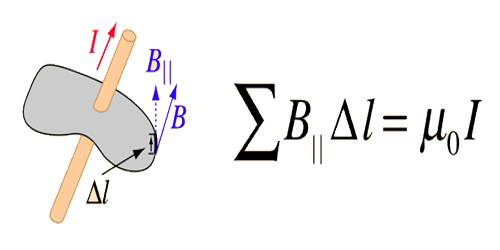Physics

# Ampere’s LawAmpere’s Law

By this law, the magnitude i.e., the magnetic field intensity produced due to the flow of current through a conductor can be determined. It establishes the relationship between the current ‘i’ flowing through that conductor and the magnetic field B produced in it. The law is as follows:

“The line integral of a magnetic field along a closed path is equal to μ0 times, the current flowing through the area subtended by that path.” The line integral of the magnetic field around some closed loop is equal to the times the algebraic sum of the currents which pass through the loop.

That means, §B.dl = μ0i … … … (1)

Here μ0 = magnetic permeability of vacuum, dl = displacement vector of path difference, § = integration in a closed path.

This law states that for any closed loop path, the sum of the length elements times the magnetic field in the direction of the length element is equal to the permeability times the electric current enclosed in the loop.